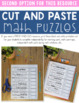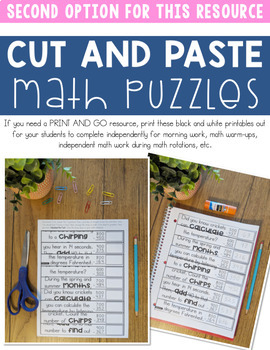# 2nd Grade Math Games | 2nd Grade Math Centers | 2nd Grade Math Puzzles | APRILSubject
Resource Type
File Type
PDF (157 MB|154 pages)
\$5.00
Also included in:
1. These 2nd grade math centers and math interactive notebook cut and paste activities focus on the 2nd grade common core math standards and are perfect for guided math rotations or math workshop!________________________________Save money, buy the 2nd Grade bundle.3rd Grade bundle.©Unravel the Fact Mat
\$42.00
\$30.00
Save \$12.00
Product Description

©Unravel the Fact Math Puzzles are an original idea by The Teacher Wears Prada.

How do these low-prep math puzzles work?

The APRIL EDITION of these cross-curricular Unravel the Fact Math Puzzles reveal facts themed around holidays in April. Students will answer the 9 math problems, put the puzzle strips in order from LEAST to GREATEST. If they have answered the problems correctly and have placed the numbers in order correctly, an interesting fact will be revealed. They will have "unraveled a fact".

Content Integration + Self-Correcting Math Puzzles = Happy Teacher and Engaged Students

What themes/facts are featured this month?

April Fool's Day, Earth Day, Facts about Planet Earth/Solar System, Caesar Chavez Day, Thomas Jefferson's Birthday, Various Spring Animals, National Garlic Day.

This resource includes 61 different math puzzles {two different formats} that cover a variety of 2nd Grade Common Core Math Skills. A recording sheet for accountability and a fact booklet for students to record their facts {optional} are also included.

Two different formats included:

Option #1: {MATH CENTERS} Print on colored paper, laminate, students write and wipe the answers with a thin dry erase marker. These laminated puzzles can be placed in math centers.

OR

Option #2: {PRINT AND GO RESOURCE} Pieces are already mixed up/jumbled up for students to solve, cut and put together to glue into math notebook or on a piece of construction paper. Students can complete these independently for morning work, math warm-ups, independent math work during math rotations, etc.

What skills are included?

{The Common Core standard is printed in the upper left corner of each puzzle}

• Telling Time (Half Hour)
• Telling Time (Quarter Hour)
• Telling Time (Five Minutes)
• Counting Money (pennies, nickels, dimes, quarters)
• Equal Parts
• Identifying Fractions
• Counting by 5s and 10s on a Number Line
• Counting by 100s
• Before and After (3 Digit Numbers)
• 3 Addends (two digits and single digit)
• Single Digit Subtraction Facts
• Mentally Add 10 (Input Table)
• Mentally Subtract 10 (Input Table)
• Mentally Add 100 (Input Table)
• Mentally Subtract 100 (Input Table)
• Even and Odd
• Plus 1, Minus 1, Plus 10, Minus 10 with 3 Digit Number
• 2 Digit Addition (Without Regrouping)
• 2 Digit Subtraction (Without Regrouping)
• 2 Digit Addition (With Regrouping)
• 2 Digit Subtraction (With Regrouping)
• 3 Digit Addition (No Regrouping)
• 3 Digit Subtraction (No Regrouping)
• Ordering Numbers (3 Digit Numbers)
• Comparing Numbers (2 Digit and 3 Digit)
• Part Part Whole
• Missing Addend (one digit and two digit)
• Bar Graph
• Pictograph
• Word Problems (Addition and Subtraction)
• TWO STEP Word Problems
• Customary Units
• Measurement (inches)
• Measurement (centimeters)
• Fact Families
• Related Facts
• Place Value
• Base Ten Blocks
• Identify the Value (3 Digit Numbers)
• Even and Odd Numbers (3 Digit Numbers)
• Word Form
• Expanded Form
• 2D Shapes
• 3D Shapes
• Multiplication (1s Facts)
• Arrays
• Regrouping Tens and Ones

___________________________________________________

Be the first to know about my new discounts, freebies and product launches:

If you're interested in more free resources, you can get access to my Free Resource Library here.

Connect with me:

Total Pages
154 pages